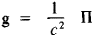# Poynting Vector

Also found in: Wikipedia.

## Poynting vector

[′pȯint·iŋ ‚vek·tər]
(electromagnetism)
A vector, equal to the cross product of the electric-field strength and the magnetic-field strength (mks units) whose outward normal component, when integrated over a closed surface, gives the outward flow of electromagnetic energy through that surface.
McGraw-Hill Dictionary of Scientific & Technical Terms, 6E, Copyright © 2003 by The McGraw-Hill Companies, Inc.
The following article is from The Great Soviet Encyclopedia (1979). It might be outdated or ideologically biased.

## Poynting Vector

the vector of the flux density of electromagnetic energy; named after the English physicist J. H. Poynting (1852–1914).

The magnitude of the Poynting vector is equal to the energy transferred per unit time through a unit of surface perpendicular to the direction of propagation of the electromagnetic energy, that is, to the direction of the Poynting vector. In the absolute (Gaussian) system of units, Π = (e/π)[EH], where [EH] is the vector product of the intensities of the electric flux E and magnetic field H and c is the speed of light in a vacuum; in the International System, Π = [EH]. The flow of the Poynting vector through a closed surface bounding a system of charged particles gives the value of the energy lost by the system per unit time as a result of the emission of electromagnetic waves. The momentum density of an electromagnetic field g is expressed in terms of the Poynting vector:G. IA. MIAKISHEV

The Great Soviet Encyclopedia, 3rd Edition (1970-1979). © 2010 The Gale Group, Inc. All rights reserved.
References in periodicals archive ?
In addition, we have a new velocity field V, which has the role of indicating the direction and speed of development of the energy flow (a sort of generalization of the Poynting vector).
The on-surface longitudinal Poynting vector of a 10-wavelength, center-fed dipole consequently also decays with distance as shown in Figure 3 .
Figure 7 demonstrates magnetic field [H.sub.z] (Figure 7(a)) and Poynting vector [P.sub.y] (Figure 7(b)) pattern as a function on [k.sub.x] and y.
First, Finite Difference Time Domain (FDTD) simulation results at known values of the implant depth are used to obtain the magnitude of the Poynting vector data at distances on and off the body surface, after a UWB signal is applied at the input of the implanted sensor antenna.
It has been obtained that a real part of the Poynting vector normal component of a wave propagating in an anisotropic medium is not equal to zero when an incident wave propagates tangentially to an interface between the media.
Using the computed electromagnetic field values, the spatial distribution of the surface current density excited on the reflector and on the dipole arms, as well as the real part of the Poynting vector, and the radiation patterns (see Figure 9) have been evaluated at the working frequencies of 5 GHz, 6 GHz, and 7 GHz, both for the antenna with and without metal reflector.
The linear momentum density is given by the Poynting vector E x B and the angular momentum is the cross product of the Poynting vector with the position vector.
To further understand the effects of LSP on different polarization of dipole source, the magnitude of Poynting vector at the wavelength of 402.7 nm and 460 nm is shown in Figures 6 and 7 in the case of [h.sub.NR] = 400 nm and [d.sub.Ag] =100 nm.
This choice can be expressed by means of the Poynting vector of (E, H) evaluated at [c.sub.n] as
[r.sub.k] = [[[u.sub.k], [v.sub.k], [w.sub.k]].sup.T] = [[sin [[theta].sub.k] cos [[phi].sub.k], sin [[theta].sub.k] sin [[phi].sub.k], cos [[theta].sub.k]].sup.T] denotes the normalized Poynting vector. [(x).sup.T] denotes the transpose.
To this end, let us consider the EM field distribution in the periodic structure and the imaginary part of the Poynting vector. It is generally known, that the Poynting energy flux vector S is a complex quantity [39, 40]

Site: Follow: Share:
Open / Close